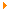Over 2000 books to read online or download to your PC, Kindle, Android, iPad, iPhone and any electronic book reader
 Find an eBook by title or author...Electronic booksEconomics and BusinessEconometrics
----------------------

 James P. LeSage eBook Edition: 1998 This text describes a set of MATLAB functions that implement a host of econometric estimation methods. Toolboxes are the name given by the MathWorks to related sets of MATLAB functions aimed at solving a particular class of problems. Toolboxes of functions useful in signal processing, optimization, statistics, _nance and a host of other areas are available from the MathWorks as add-ons to the standard MATLAB software distribution. The author use the term Econometrics Toolbox to refer to the collection of function libraries described in this book. eBook Contents Preface – Introduction - Regression using MATLAB - Utility Functions - Regression Diagnostics - VAR and Error Correction Models - Markov Chain Monte Carlo Models - Limited Dependent Variable Models - Simultaneous Equation Models - Distribution functions library - Optimization functions library - Handling sparse matrices – References - Appendix eBook License: Copyrighted (Personal Use Only) Free PDF eBook - 1.45 Mb - 332 pages